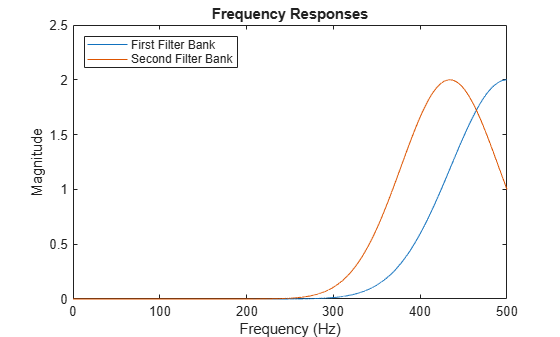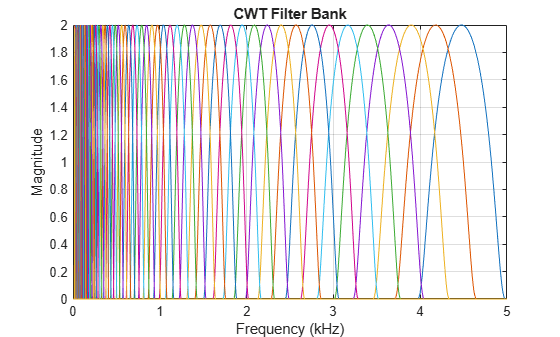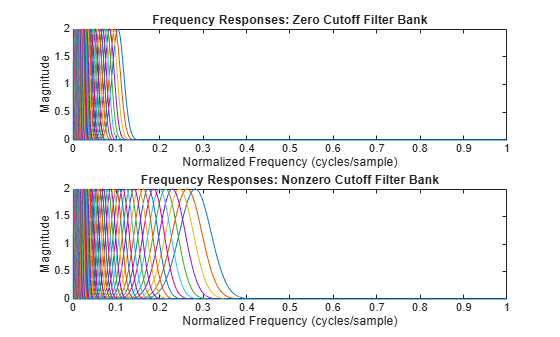# cwtfreqbounds

CWT maximum and minimum frequency or period

## Syntax

``[minfreq,maxfreq] = cwtfreqbounds(N)``
``[minfreq,maxfreq] = cwtfreqbounds(N,Fs)``
``[maxperiod,minperiod] = cwtfreqbounds(N,Ts)``
``[___] = cwtfreqbounds(___,Name=Value)``

## Description

example

````[minfreq,maxfreq] = cwtfreqbounds(N)` returns the minimum and maximum wavelet bandpass frequencies in cycles/sample for a signal of length `N`. The minimum and maximum frequencies are determined for the default Morse (3,60) wavelet. The minimum frequency is determined so that two time standard deviations of the default wavelet span the `N`-point signal at the coarsest scale. The maximum frequency is such that the highest frequency wavelet bandpass filter drops to ½ of its peak magnitude at the Nyquist frequency.```
````[minfreq,maxfreq] = cwtfreqbounds(N,Fs)` returns the bandpass frequencies in hertz for the sampling frequency `Fs`.```
````[maxperiod,minperiod] = cwtfreqbounds(N,Ts)` returns the bandpass periods for the sampling period `Ts`. `maxperiod` and `minperiod` are scalar durations with the same format as `Ts`. If the number of standard deviations is set so that `log2(maxperiod/minperiod) < 1/NV` where `NV` is the number of voices per octave, `maxperiod` is adjusted to ```minperiod × 2^(1/NV)```.```

example

````[___] = cwtfreqbounds(___,Name=Value)` returns the minimum and maximum wavelet bandpass frequencies or periods with additional options specified by one or more `Name=Value` arguments. For example, ```[minf,maxf] = cwtfreqbounds(1000,TimeBandwidth=30)``` sets the time-bandwidth parameter of the default Morse wavelet to 30.```

## Examples

collapse all

Obtain the minimum and maximum wavelet bandpass frequencies for a signal with 1000 samples using the default values.

`[minfreq,maxfreq] = cwtfreqbounds(1000)`
```minfreq = 0.0033 ```
```maxfreq = 0.4341 ```

Obtain the minimum and maximum wavelet bandpass frequencies for the default Morse wavelet for a signal of length 10,000 and a sampling frequency of 1 kHz. Set the cutoff to 100% so that the highest frequency wavelet bandpass filter peaks at the Nyquist frequency of 500 Hz.

```sigLength = 10000; Fs = 1e3; [minfreq,maxfreq] = cwtfreqbounds(sigLength,Fs,cutoff=100);```

Construct a CWT filter bank using the values `cwtfreqbounds` returns. Obtain the frequency responses of the filter bank.

```fb = cwtfilterbank(SignalLength=sigLength,SamplingFrequency=Fs,... FrequencyLimits=[minfreq maxfreq]); [psidft,f] = freqz(fb);```

Construct a second CWT filter bank identical to the first, but instead use the default frequency limits. Obtain the frequency responses of the second filter bank.

```fb2 = cwtfilterbank(SignalLength=sigLength,SamplingFrequency=Fs); [psidft2,~] = freqz(fb2);```

For each filter bank, plot the frequency response of the filter with the highest center frequency. Confirm the frequency response from the first filter bank peaks at the Nyquist, and the frequency response from the second filter bank is 50% of the peak magnitude at the Nyquist.

```plot(f,psidft(1,:)) hold on plot(f,psidft2(1,:)) hold off title("Frequency Responses") xlabel("Frequency (Hz)") ylabel("Magnitude") legend("First Filter Bank","Second Filter Bank",... Location="NorthWest")```Obtain the minimum and maximum frequencies for the bump wavelet for a signal of length 5,000 and a sampling frequency of 10 kHz. Specify a cutoff value of $100×1{0}^{-8}/2$ so that the highest frequency wavelet bandpass filter decays to $1{0}^{-8}$ at the Nyquist.

`[minf,maxf] = cwtfreqbounds(5e3,1e4,wavelet="bump",cutoff=100*1e-8/2);`

Construct the filter bank using the values returned by `cwtfreqbounds`. Plot the frequency responses.

```fb = cwtfilterbank(SignalLength=5e3,Wavelet="bump",... SamplingFrequency=1e4,FrequencyLimits=[minf maxf]); freqz(fb)```Obtain the minimum and maximum wavelet bandpass frequencies for a signal of length 4096. Specify a cutoff of 0. Display the minimum and maximum bandpass frequencies.

```sLength = 4096; co = 0; [minfreq,maxfreq] = cwtfreqbounds(sLength,Cutoff=co); fprintf("Min Frequency: %f cycles/sample\nMax Frequency: %f cycles/sample", ... minfreq,maxfreq)```
```Min Frequency: 0.000805 cycles/sample Max Frequency: 0.103574 cycles/sample ```

Create a filter bank using the frequency limits. Obtain the two-sided wavelet frequency responses.

```fb = cwtfilterbank(SignalLength=sLength,FrequencyLimits=[minfreq,maxfreq]); [psif,f] = freqz(fb,FrequencyRange="twosided");```

Obtain the minimum and maximum wavelet bandpass frequencies for a signal of length 4096, but this time specify a cutoff of $100×1{0}^{-8}/2$. Create a second filter bank using these new frequencies. Confirm the second frequency range is larger than the first frequency range.

```co = 100*(1e-8/2); [minfreq2,maxfreq2] = cwtfreqbounds(sLength,Cutoff=co); fb2 = cwtfilterbank(SignalLength=sLength,FrequencyLimits=[minfreq2,maxfreq2]); fprintf("Min Frequency: %f cycles/sample\nMax Frequency: %f cycles/sample", ... minfreq2,maxfreq2);```
```Min Frequency: 0.000805 cycles/sample Max Frequency: 0.281770 cycles/sample ```

Obtain the two-sided wavelet frequency responses of the second filter bank.

`[psif2,f2] = freqz(fb2,FrequencyRange="twosided");`

Plot the frequency responses of the filter banks.

```subplot(2,1,1) plot(f,psif) title("Frequency Responses: Zero Cutoff Filter Bank") ylabel("Magnitude") xlabel("Normalized Frequency (cycles/sample)") subplot(2,1,2) plot(f2,psif2) title("Frequency Responses: Nonzero Cutoff Filter Bank") ylabel("Magnitude") xlabel("Normalized Frequency (cycles/sample)")```For the wavelet filter with the highest center frequency in each filter bank, obtain the magnitude of the frequency response at the Nyquist. Observer there is minimal difference between the two values.

`fprintf("Zero Cutoff / Magnitude at Nyquist: %g",psif(1,floor(size(psif,2)/2)))`
```Zero Cutoff / Magnitude at Nyquist: 2.43333e-309 ```
`fprintf("Nonzero Cutoff / Magnitude at Nyquist: %g",psif2(1,floor(size(psif2,2)/2)))`
```Nonzero Cutoff / Magnitude at Nyquist: 1.02265e-08 ```

## Input Arguments

collapse all

Signal length, specified as a positive integer greater than or equal to 4.

Data Types: `double`

Sampling frequency in hertz, specified as a positive scalar.

Example: ```[minf,maxf] = cwtfreqbounds(2048,100)```

Data Types: `double`

Sampling period, specified as a positive scalar `duration`.

Example: ```[minp,maxp] = cwtfreqbounds(2048,seconds(2))```

Data Types: `duration`

### Name-Value Arguments

Specify optional pairs of arguments as `Name1=Value1,...,NameN=ValueN`, where `Name` is the argument name and `Value` is the corresponding value. Name-value arguments must appear after other arguments, but the order of the pairs does not matter.

Example: ```[minf,maxf] = cwtfreqbounds(1000,Wavelet="bump",VoicesPerOctave=10)``` returns the minimum and maximum bandpass frequencies using the bump wavelet and 10 voices per octave for a signal with 1000 samples.

Before R2021a, use commas to separate each name and value, and enclose `Name` in quotes.

Example: ```[minf,maxf] = cwtfreqbounds(1000,"Wavelet","bump","VoicesPerOctave",10)```

Analysis wavelet used to determine the minimum and maximum frequencies or periods, specified as `"Morse"`, `"amor"`, or `"bump"`. These strings specify the analytic Morse, Morlet, and bump wavelet, respectively. The default wavelet is the analytic Morse (3,60) wavelet.

For Morse wavelets, you can also parametrize the wavelet using the `TimeBandwidth` or `WaveletParameters` name-value arguments.

Example: ```[minp,maxp] = cwtfreqbound(2048,seconds(1),Wavelet="bump")```

Percentage of the peak magnitude at the Nyquist, specified as a scalar between 0 and 100. Setting `Cutoff` to `0` indicates that the wavelet frequency response decays to 0 at the Nyquist. Setting `Cutoff` to `100` indicates that the value of the wavelet bandpass filters peaks at the Nyquist.

For `cwtfilterbank`, the analytic wavelets filters peak at a value of 2. As a result, you can ensure the highest frequency wavelet decays to a value of α at the Nyquist frequency by setting `Cutoff` to 100 × α/2. In that case, you must have 0 ≤ α ≤ 2.

Note

Unless your application requires a strict cutoff value of 0, consider setting `Cutoff` to a small nonzero value, for example, on the order of 10-8. By specifying a small value, you can increase the frequency range `[minfreq,maxfreq]` and still obtain a wavelet frequency response that effectively decays to 0 at the Nyquist. See Frequency Range for Strictly Zero and Effectively Zero Cutoff Values.

Data Types: `double`

Number of time standard deviations used to determine the minimum frequency (longest scale), specified as a positive integer greater than or equal to 2. For the Morse, analytic Morlet, and bump wavelets, four standard deviations generally ensures that the wavelet decays to zero at the ends of the signal support. Incrementing `StandardDeviations` by multiples of 4, for example 4*M, ensures that M whole wavelets fit within the signal length. If the number of standard deviations is set so that ```log2(minfreq/maxfreq) > -1/NV```, where `NV` is the number of voices per octave, `minfreq` is adjusted to ```maxfreq × 2^(-1/NV)```.

Data Types: `double`

Time-bandwidth for the Morse wavelet, specified as a positive scalar. The symmetry (gamma) of the Morse wavelet is assumed to be 3. The larger the time-bandwidth parameter, the more spread out the wavelet is in time and narrower the wavelet is in frequency. The standard deviation of the Morse wavelet in time is approximately `sqrt(TimeBandwidth/2)`. The standard deviation in frequency is approximately `1/2*sqrt(2/TimeBandwidth)`.

If you specify `TimeBandwidth`, you cannot specify `WaveletParameters`.

Data Types: `double`

Morse wavelet parameters, specified as a two-element vector. The first element is the symmetry parameter (gamma), which must be greater than or equal to 1. The second element is the time-bandwidth parameter, which must be greater than or equal to gamma. The ratio of the time-bandwidth parameter to gamma cannot exceed 40.

When gamma is equal to 3, the Morse wavelet is perfectly symmetric in the frequency domain. The skewness is equal to 0. Values of gamma greater than 3 result in positive skewness, while values of gamma less than 3 result in negative skewness.

If you specify `WaveletParameters`, you cannot specify `TimeBandwidth`.

Data Types: `double`

Number of voices per octave to use in determining the necessary separation between the minimum and maximum scales, specified as an integer between 1 and 48. The minimum and maximum scales are equivalent to the minimum and maximum frequencies or maximum and minimum periods, respectively.

Data Types: `double`

## Output Arguments

collapse all

Minimum wavelet bandpass frequency, returned as a scalar. `minfreq` is in cycles/sample if `SamplingFrequency` is not specified. Otherwise, `minfreq` is in hertz.

Data Types: `double`

Maximum wavelet bandpass frequency, returned as a scalar. `maxfreq` is in cycles/sample if `SamplingFrequency` is not specified. Otherwise, `maxfreq` is in hertz.

Data Types: `double`

Maximum wavelet bandpass period, returned as a scalar duration with the same format as `Ts`.

If the number of standard deviations is set so that `log2(maxperiod/minperiod) < 1/NV`, where `NV` is the number of voices per octave, `maxperiod` is adjusted to ```minperiod × 2^(1/NV)```.

Data Types: `duration`

Minimum wavelet bandpass period, returned as a scalar duration with the same format as `Ts`.

If the number of standard deviations is set so that `log2(maxperiod/minperiod) < 1/NV`, where `NV` is the number of voices per octave, `maxperiod` is adjusted to ```minperiod × 2^(1/NV)```

Data Types: `duration`

## Version History

Introduced in R2018a